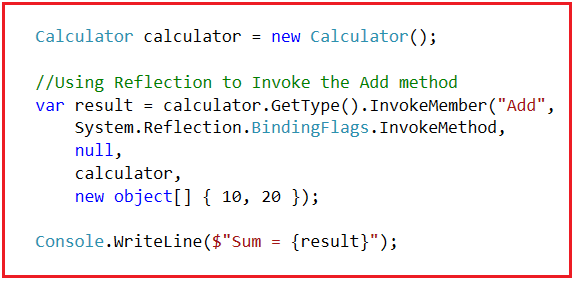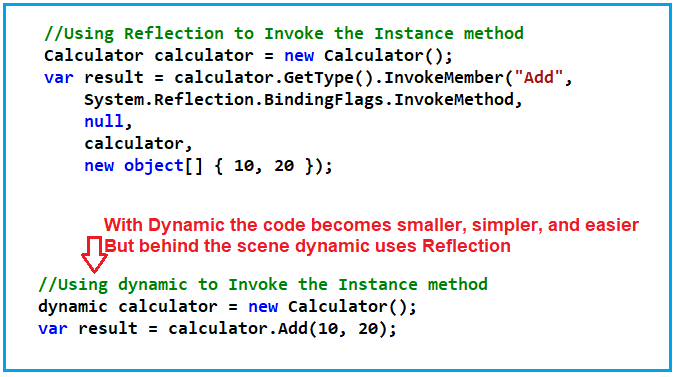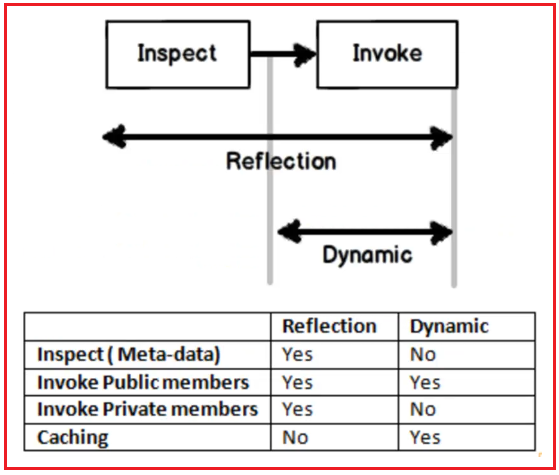# Dynamic vs Reflection in C#

## Dynamic vs Reflection in C# with Examples

In this article, I am going to discuss Dynamic vs Reflection in C# with Examples. Please read our Dynamic in C# and Reflection in C# articles before proceeding with this article where we explained the concept of Dynamic and Reflection in detail. Here, in this article, I am not going to explain what is dynamic and what is Reflection rather I will focus on discussing the differences between dynamic and reflection in C#.

##### Dynamic vs Reflection in C#:

Let us understand the differences between Dynamic vs Reflection in C# with an example. With dynamic in C#, it is very easy to write reflection code which in turn makes the code more readable and maintainable.

Let us see an example for a better understanding. We want to invoke an instance method, first using reflection, and then we will invoke the same instance method using dynamic in C#. Please have a look at the following Calculator class.

```public class Calculator
{
public int Add(int number1, int number2)
{
return number1 + number2;
}
}
```

This is a very simple class. This class had one method i.e. Add which takes two integer parameters and this method then returns the sum of the two input numbers. Now, we want to invoke the above Add method using both Reflections as well as Dynamic. Let us first invoke the above Add method using Reflection. The following example code shows how to invoke the above Add method using Reflection in C#.You can observe the number of codes we need to write in order to invoke the Add method of the Calculator class using Reflection in C#. The complete example is given below.

```using System;
namespace DynamicVSReflectionDemo
{
class Program
{
static void Main(string[] args)
{
Calculator calculator = new Calculator();

//Using Reflection to Invoke the Add method
System.Reflection.BindingFlags.InvokeMethod,
null,
calculator,
new object[] { 10, 20 });

Console.WriteLine(\$"Sum = {result}");
}
}

public class Calculator
{
public int Add(int number1, int number2)
{
return number1 + number2;
}
}
}
```

Output: Sum = 30

Here, as you can see, we have written a lot of code just to call the Add method using Reflection. The code size is not only big but also it is quite complex as well as difficult to understand. The above reflection code can be rewritten using dynamic in C#. Using dynamic, the code will be simpler, cleaner, and easier to understand. The following example shows how to use dynamic to invoke the Add method of the Calculator class.

```using System;
namespace DynamicVSReflectionDemo
{
class Program
{
static void Main(string[] args)
{
//Using dynamic to Invoke the Add method
dynamic calculator = new Calculator();
Console.WriteLine(\$"Sum = {result}");
}
}

public class Calculator
{
public int Add(int number1, int number2)
{
return number1 + number2;
}
}
}
```

Output: Sum = 30

##### Differences between Reflection and Dynamic in C#:

The first and foremost difference is that using dynamic is very simple, less code, and easily understandable as compared with the reflection. For a better understanding, please have a look at the below image which shows how to invoke an instance method using Reflection and Dynamic in C#.Both dynamic and reflection use dynamic invocation. So, we should understand in what scenarios we need to use dynamic and in what other scenarios we need to use reflection. For this to understand, please have a look at the following diagram.The first point that you need to remember is the dynamic keyword internally uses Reflection Framework. Reflection does two things. First, it inspects the metadata. That is, it determines what are the methods, properties, and fields of the assembly. And the second thing is that, using reflection we can also invoke those methods, properties, fields, etc. dynamically. On the other hand, the dynamic keyword only does invocation, it does not perform the inspection.

So, the biggest difference between dynamic and reflection is that if you are talking about inspecting metadata, then you should use Reflection. But if you are invoking the methods, properties, fields, etc, of an object, then you need to use the dynamic keyword in C#.

1. Inspect Metadata: Reflection can inspect the metadata but dynamic can’t inspect the metadata of an assembly.
2. Invoking Public Members: We can invoke the public members of an object using both reflection and dynamic. It is recommended to use dynamic because of its simplicity and easy-to-use feature.
3. Invoking Private Members: We can invoke the private members of an object using reflection but cannot invoke the private members of an object using dynamic.
4. Caching: We can cache using dynamic but not with reflection.

Note: So, use reflection when you want to inspect the metadata and when you want to invoke the private members. Use dynamic when you want to invoke the public members and when you want to use caching.

1.